# 10-327/Classnotes for Thursday November 18

See some blackboard shots at BBS/10_327-101118-142430.jpg (unfortunately some blackboards from the middle of the proof or Urysohn's lemma got forgotten).

 Dror's notes above / Student's notes below

Here are some lecture notes:

Questions by Kai Xwbdsb 21:26, 19 November 2010 (EST):

1. I have concern about this "Adding T_1 thing". When we are proving the Urysohn's Lemma, we proved that if X is a T.S. and A,B are any two disjoint closed sets in X, they can be separated by two disjoint open sets in X iff they can be separated by a continuous function. So there is no T_1 axiom involved in any of these two proofs. So Urysohn's Lemma is something more general than T_4 iff T_4.5 right?
• You are probably right and Urysohn applies even without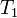$T_1$. Yet we want$T1$ all else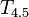$T_{4.5}$ does not imply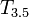$T_{3.5}$ and below, and we will need$T_{3.5}$ very soon. Drorbn 06:02, 20 November 2010 (EST)
2. I just want to also confirm the definition of T_4 and T_4.5: T_4 is T_1 + separation by two disjoint open sets for any two disjoint closed sets and T_4.5 is T_1 + separation by a continuous function for any two disjoint closed sets?
• Indeed so. Drorbn 06:02, 20 November 2010 (EST)
3. The only purpose of this manually adding T_1 thing is that so that T_4 and T_4.5 could imply T_3 T_2 T_1 right?
• Indeed. Drorbn 06:02, 20 November 2010 (EST)
4. Dror you said before that induction can go up to finite n but why in this case induction will work for a countably infinite set of elements?
• We are never making a statement about an infinite set of rational numbers directly through induction. We are only constructing something for the$n$'th rational, for each$n$. We then look at the totality of these constructions, but that's already outside the induction. Drorbn 06:02, 20 November 2010 (EST)

Kai: So you mean if properly used, mathematical induction could prove things involve countably infinite objects right? Just like in this case. -KaiXwbdsb 11:32, 20 November 2010 (EST)

John: Induction can be hard to understand, what induction bascially says is; If Property P holds for 1, and P holds for k implies P holds for k+1 then P holds for all natural numbers. So the property holds "with respect to" an a infinite coutable set (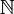$\mathbb{N}$) but this does not mean the property holds "for" infinite cardinals. So something like, The finite intersection of open sets is open could use an inductive prove the intersection of one open set is open and the intersection of k open sets is open implies the intersection of k+1 open sets is open, then the intersection of any natural number of open sets is open. But of course infinite intersections of open sets are not necessarily open. So in this case there are an infinite number of ways to take intersections, but we cannot use infinite intersections themselves. In the proof done in class, we basically said, take a set with the first n rational numbers (giving by some ordering we choose) that has the required construction then we prove we can construct something for the set with the first n+1 rational numbers. So we have the construction that works for any set that has the cardinality of a natural number, but the construction does not work for a set with an infinite cardinality.

I don't know if this helps, it took me a while to grasp this idea and it's how I think about it. -John

Dror: Induction allows you to prove something for every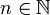$n\in{\mathbb N}$, or to construct something for every$n\in{\mathbb N}$, or if you enumerate the elements of a countable set, induction will allow you to do the same for every element of that countable set. So induction in some sense always does something infinite, as it proves/constructs something for every member of an infinite set like${\mathbb N}$ or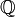${\mathbb Q}$. Yet induction says nothing about "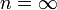$n=\infty$". I don't know what "$n=\infty$" is, in general. "$n=\infty$" is not a member of${\mathbb N}$ or${\mathbb Q}$. And if in some specific inductive argument$n$ refers to the cardinality of a set$A$, then at the end of the inductive proof you would know that a certain thing has been proven for every finite size$n$ (and there are infinitely many such finite$n$'s), yet you would know nothing about the case where$A$ itself is infinite. (Of course, sometimes you'd be able to prove something about infinite$A$'s as well, but that would be an additional argument, beyond the induction). Drorbn 15:09, 20 November 2010 (EST)

• Thanks John and Dror for the explanation. It is very tricky. I believe I have grasped your ideas? For each member in${\mathbb Q}$, we can find its position in the list which is a finite number and here is where induction applies. After that we look from "outside" of the induction we have done the construction for every member in${\mathbb Q}$. Basically we did construction on a infinite set but that is already "outside" of induction. -Kai Xwbdsb 17:11, 20 November 2010 (EST)
• I agree. Drorbn 06:38, 22 November 2010 (EST)